Basic Ammeter Use

Basic Electricity

• Question 1

Show how this ammeter would be connected to the light bulb circuit to measure the circuit’s electric current: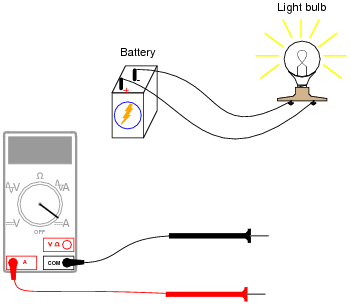Also, draw a schematic diagram of this same circuit (with the ammeter connected).

• Question 2

Shown here is a circuit constructed on a PCB (a “Printed Circuit Board”), with copper “traces” serving as wires to connect the components together: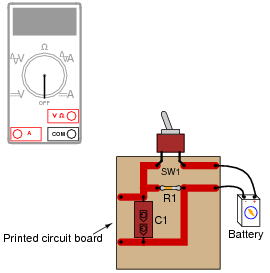How would the multimeter be used to measure the current through the component labeled “R1” when energized? Include these important points in your answer:

• The configuration of the multimeter (selector switch position, test lead jacks)
• The connections of the meter test leads to the circuit
• The state of the switch on the PCB (open or closed)
• Question 3

Why is it a very bad idea to connect an ammeter directly across a voltage source, like this?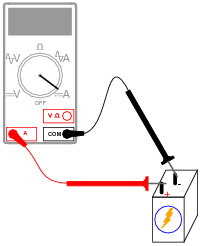• Question 4

What would happen if a multimeter were connected across the component labeled “C1” on this printed circuit board, as shown?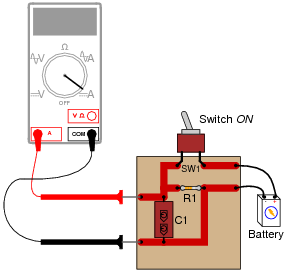• Question 5

If we were to connect an ammeter directly to an ohmmeter, what would you expect to see the ohmmeter register, for resistance between its test leads?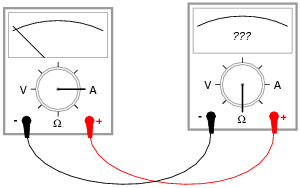• Question 6

Most ammeters contain fuses inside to provide protection for the person using the ammeter, as well as for the ammeter mechanism itself. Voltmeters generally do not contain fuses inside, because they are unnecessary.

Explain why ammeters use fuses and voltmeters do not? What is it about the nature of an ammeter and how it is used that makes fuse-protection necessary?

• Question 7

In this circuit, is the light bulb lit? Why or why not?

Also, compare the relative indications of the two ammeters (which ammeter registers the greatest amount of current, and which ammeter registers the least amount of current, or do they both register the same amount of current?).• Question 8

In this circuit, is the light bulb lit? Why or why not?

Also, compare the relative indications of the two ammeters (which ammeter registers the greatest amount of current, and which ammeter registers the least amount of current, or do they both register the same amount of current?).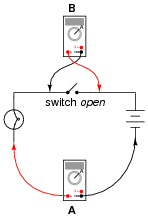• Question 9

In this circuit, is the light bulb lit? Why or why not?

Also, compare the relative indications of the two ammeters (which ammeter registers the greatest amount of current, and which ammeter registers the least amount of current, or do they both register the same amount of current?).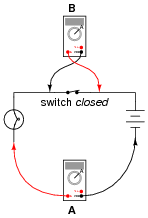• Question 10

Generally speaking, is an ammeter supposed to be connected in series or in parallel with the component it’s measuring current through?

• Question 11

Ammeters must be connected in series with the current to be measured, to ensure that all the current moves through the meter:In order to practically function, an ammeter must have some internal resistance. It is usually a very small amount, but it does exist. It should be apparent to you that the presence of this resistance will have some effect on the circuit current, when compared to the amount of current in the circuit without any meter connected:Explain why it is usually safe to ignore the internal resistance of an ammeter, though, when it is in a circuit. A common term used in electrical engineering to describe this intentional oversight is swamping. In this particular circuit an engineer would say, “The resistance of the light bulb swamps the internal resistance of the ammeter.”